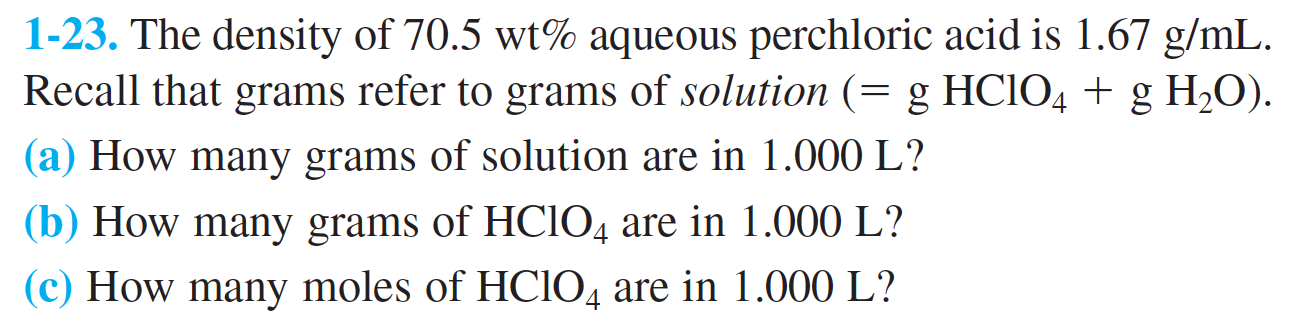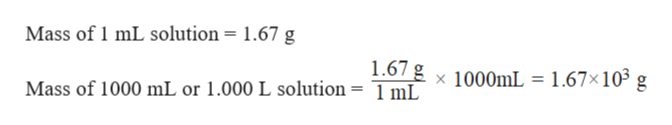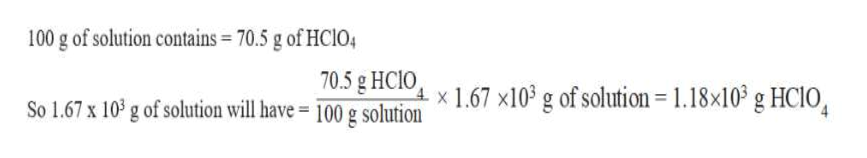# 1-23. The density of 70.5 wt% aqueous perchloric acid is 1.67 g/mLRecall that grams refer to grams of solution (= g HCIO4 g H2O)|(a) How many grams of solution are in 1.000 L?|(b) How many grams of HCIO4 are in 1.000 L?(c) How many moles of HCIO4 are in 1.000 L?

Question
90 viewshelp_outlineImage Transcriptionclose1-23. The density of 70.5 wt% aqueous perchloric acid is 1.67 g/mL Recall that grams refer to grams of solution (= g HCIO4 g H2O) |(a) How many grams of solution are in 1.000 L? |(b) How many grams of HCIO4 are in 1.000 L? (c) How many moles of HCIO4 are in 1.000 L? fullscreen
check_circle

Step 1

Density is a physical quantity that can be determined with the help of the mass and volume of the given substance.

The formula of the density of any substance can be written as:

Step 2

a) Calculate the mass of solution in 1.000 L

Density of solution = 1.67 g/mL

1.000 L  =  1000 mL

Hence the mass of 1.000 L solution:help_outlineImage TranscriptioncloseMass of 1 mL solution = 1.67 g 1.67 g x 1000mL = 1.67x105 g Mass of 1000 mL or 1.000 L solution = 1 mL| fullscreen
Step 3

b)  Calculate the mass of acid in 1.000 L of solution:

Weight percent of a solute is equal to the mass of the solute divided by the t...help_outlineImage Transcriptionclose100 g of solution contains 70.5 g of HCI04 70.5 g HCIO So 1.67 x 10 g of solution will have - 100 g solutionx 1.67 x10* g of solution = 1.18x103 g HC10, fullscreen

### Want to see the full answer?

See Solution

#### Want to see this answer and more?

Solutions are written by subject experts who are available 24/7. Questions are typically answered within 1 hour.*

See Solution
*Response times may vary by subject and question.
Tagged in

### Solutions